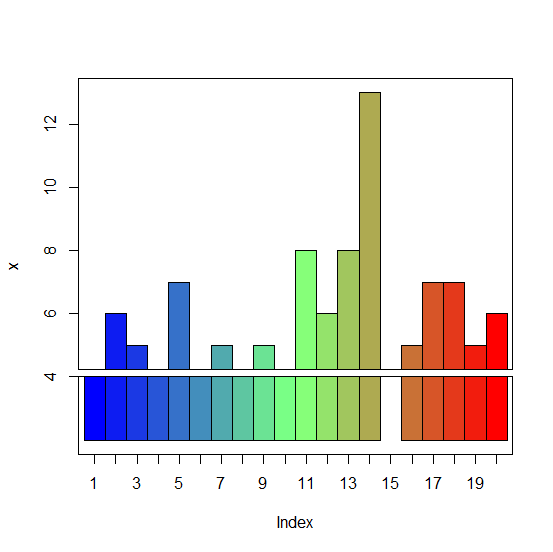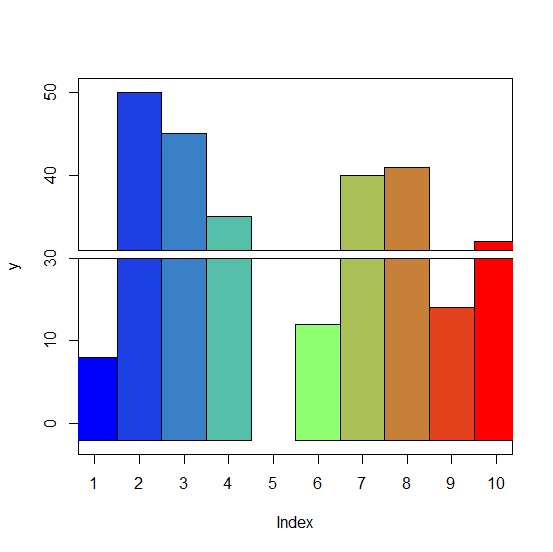# How to create a barplot with gaps on Y-axis scale in R?

If we want to have gaps on Y-axis scale in a barplot then it cannot be done in base R. For this purpose, we can make use of gap.barplot function of plotrix package. The gap.barplot function is very handy, we just need to pass the vector for which we want to create the barplot and the gap values simply by using gap argument.

> library(plotrix)

## Example1

Live Demo

> x<-rpois(20,5)
> x

## Output

 2 6 5 4 7 2 5 2 5 2 8 6 8 13 3 5 7 7 5 6

> gap.barplot(x,gap=c(2,4))
ylim 0 11

Warning message:

In gap.barplot(x, gap = c(2, 4)) : gap includes some values of y

## Output:## Example2

Live Demo

> y<-sample(0:50,10)
> y

## Output

 8 50 45 35 25 12 40 41 14 32

> gap.barplot(y,gap=c(20,30))
ylim -2 40

Warning message:

In gap.barplot(y, gap = c(20, 30)) : gap includes some values of y

## Output: